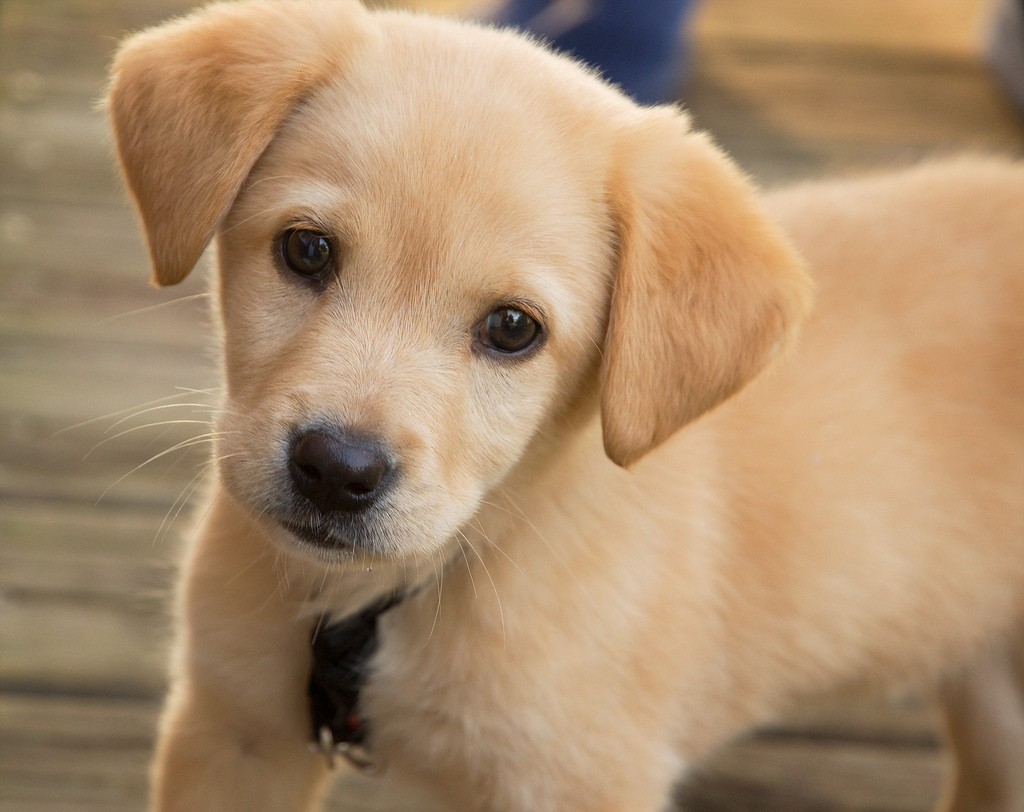#### deeplearning / SubredditClassifier / 0.1.3## Introduction

This algorithm detects which subreddit an image probably belongs to, and returns class pairs for the Top 3 best guesses.

The classifier is a Convolutional Neural Network, trained to detect the subreddit that an image belongs to; this was done using the following torch model:

``````nn.Sequential {
[input -> (1) -> (2) -> (3) -> (4) -> (5) -> (6) -> (7) -> (8) -> (9) -> (10) -> (11) -> (12) -> (13) -> (14) -> output]
(1): nn.SpatialConvolution(3 -> 6, 5x5)
(2): nn.ReLU
(3): nn.SpatialMaxPooling(2x2, 2,2)
(4): nn.SpatialConvolution(6 -> 16, 5x5)
(5): nn.ReLU
(6): nn.SpatialMaxPooling(2x2, 2,2)
(7): nn.View(55696)
(8): nn.Linear(55696 -> 1024)
(9): nn.Tanh
(10): nn.Dropout(0.125000)
(11): nn.Linear(1024 -> 120)
(12): nn.Tanh
(13): nn.Linear(120 -> 5)
(14): nn.LogSoftMax
}
``````

The above model uses multiple convolution and max pooling layers to allow the CNN model to break up the large `(3 colour channels)*height*weight` tensor into multiple smaller subregions that can each have different operations performed.

The Convolutions allows the network to create feature maps from an image, which allows the rest of the network to predict image content from extracted features instead of raw image data.

The CNN model was trained with the following image based subreddits:

## I/O

Input

`src: String`

• src - (required) - the source image to classify, this must be in either `jpg` or `png` format, mage Data API Url' Web (http/https) Url /binary image or a base64 encoded JPEG String.

Output

``````{
"first": {
"className": String,
"confidence": Float
},
"second": {
"className": String,
"confidence": Float
},
"third": {
"className": String,
"confidence": Float
}
}
``````
• first - the source image to classify, this must be in either `jpg` or `png` format, mage Data API Url' Web (http/https) Url /binary image or a base64 encoded JPEG String.
• className - The classname for the label (string).
• confidence - The confidence that this label is correct (float).

## Examples

Example 1Input

``````"http://lovelace-media.imgix.net/uploads/191/0f832b40-d622-0132-cea9-0e01949ad350.jpg"
``````

Output

``````{
"first": {
"className": "dogpictures",
"confidence": 0.9969158
},
"second": {
"className": "catpictures",
"confidence": 0.0027667093
},
"third": {
"className": "foodporn",
"confidence": 0.00031607767
}
}
``````

Example 2Input

``````"https://i.imgur.com/nraWKPw.jpg"
``````

Output

``````{
"first": {
"className": "foodporn",
"confidence": 0.99819106
},
"second": {
"className": "dogpictures",
"confidence": 0.0013163951
},
"third": {
"className": "catpictures",
"confidence": 0.00039565665
}
}
``````

Contents